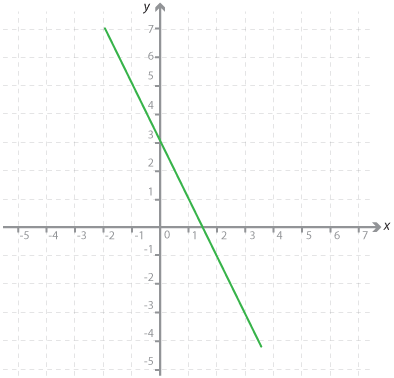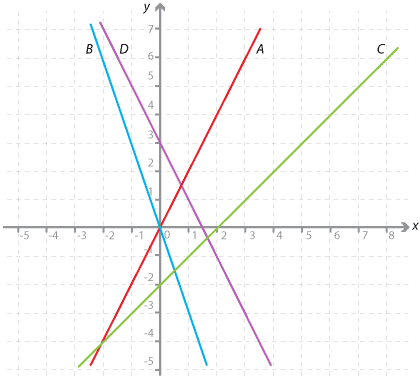#### Question 1The y-intercept of the graph of y = –2x + 3 is:

 1.5 3 –2

#### Question 2

Find the gradient of each of the following intervals:

a Interval OB where the coordinates of B are (6, 12). Interval OC where the coordinates of C are (12, 6). (Give your answer as a decimal.) Interval OD where the coordinates of D are (–6, 18). Interval MN where the coordinates of M are (1, 5) and the coordinates of N are (2, 11).

#### Question 3Detailed description

a The gradient of line A is: The gradient of line B is: The gradient of line C is: The gradient of line D is: